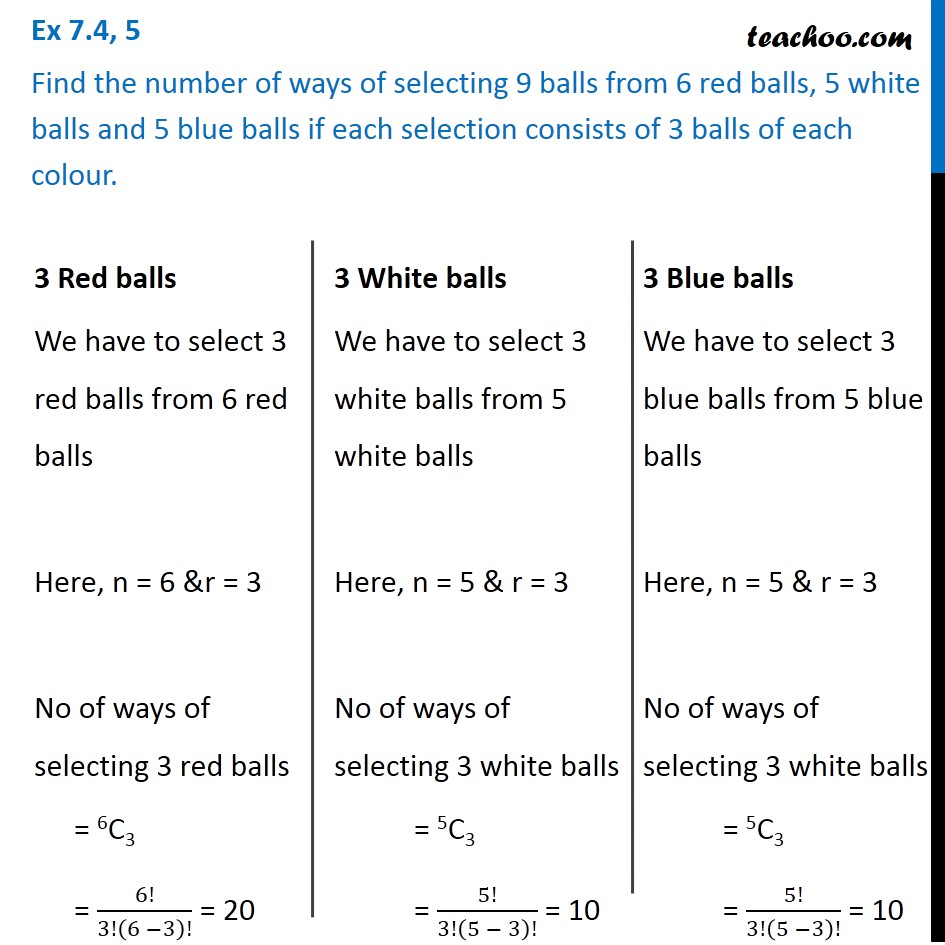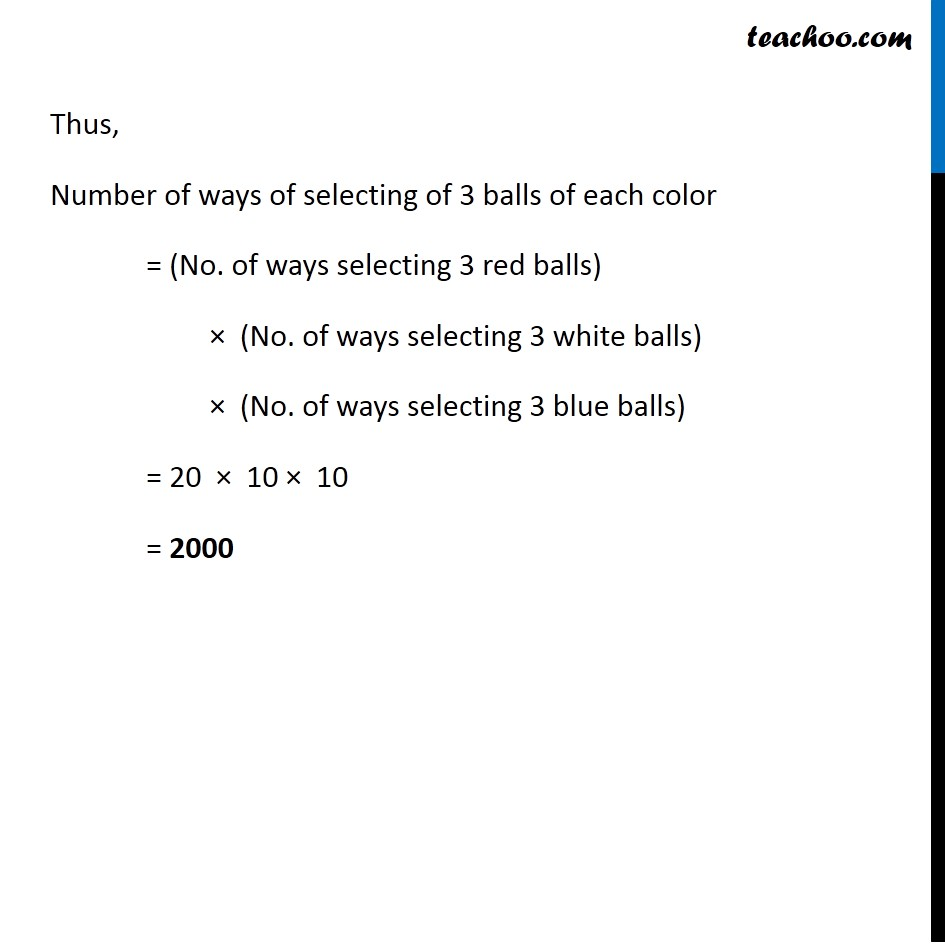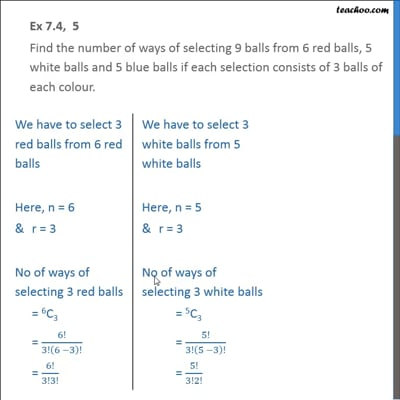Ex 7.4

Chapter 7 Class 11 Permutations and Combinations
Serial order wiseThis video is only available for Teachoo black users

Introducing your new favourite teacher - Teachoo Black, at only ₹83 per month

### Transcript

Ex 7.4, 5 Find the number of ways of selecting 9 balls from 6 red balls, 5 white balls and 5 blue balls if each selection consists of 3 balls of each colour. 3 Red balls We have to select 3 red balls from 6 red balls Here, n = 6 &r = 3 No of ways of selecting 3 red balls = 6C3 = 6!/3!(6 −3)! = 20 3 White balls We have to select 3 white balls from 5 white balls Here, n = 5 & r = 3 No of ways of selecting 3 white balls = 5C3 = 5!/3!(5 − 3)! = 10 3 Blue balls We have to select 3 blue balls from 5 blue balls Here, n = 5 & r = 3 No of ways of selecting 3 white balls = 5C3 = 5!/3!(5 −3)! = 10 Thus, Number of ways of selecting of 3 balls of each color = (No. of ways selecting 3 red balls) × (No. of ways selecting 3 white balls) × (No. of ways selecting 3 blue balls) = 20 × 10 × 10 = 2000